Data Science
Resource

# Learn Linear Algebra for Data Science

Linear Algebra is a vital cog in data scientists’ skill set that learners don't bother much about because they find maths intimidating.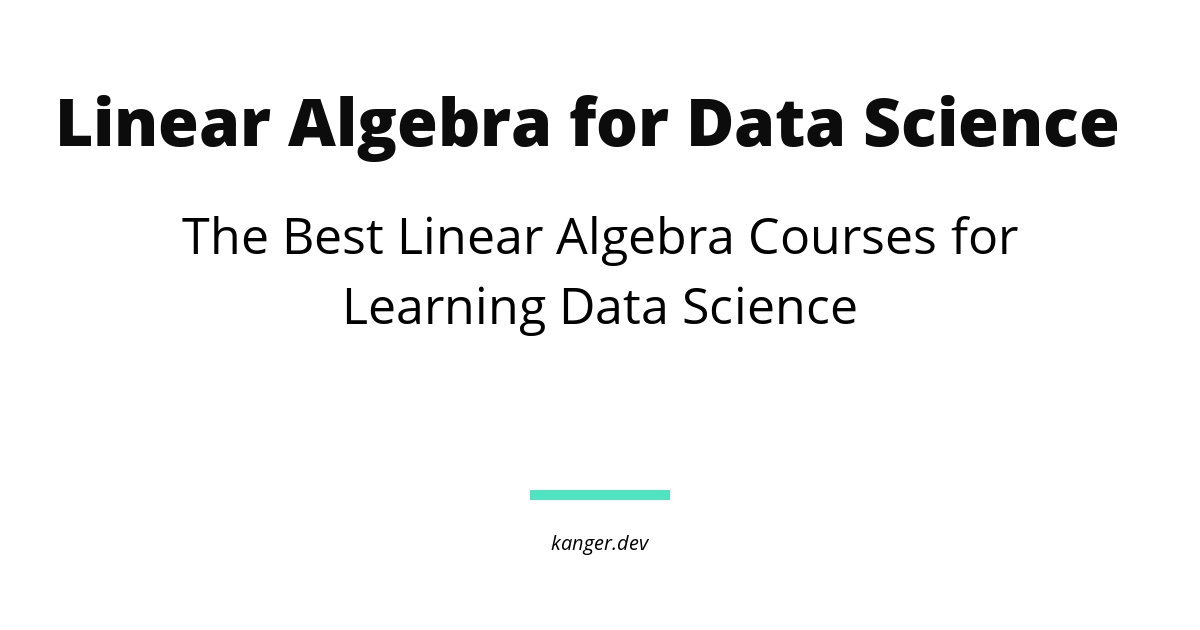Written by
Editors
Updated on
May 12, 2023

Linear Algebra is one of the foundational blocks of Data Science and Machine Learning. It’s hard to think of algorithms or applications that aren’t implemented using linear algebra.

Programming languages like Python, R and Julia offer a plethora of packages for working with data to solve complex problems by applying linear algebra. You'll be using linear algebra to perform the required calculations and analyses efficiently. It is used for Principal Component Analysis to reduce the dimensionality of data. It is practically good for working with neural networks, the representation and processing of the network.

With a functional knowledge of Linear Algebra, you will develop a better intuition for ML and deep learning algorithms. It is your key to unlock a whole new world — you will also be able to code algorithms from scratch and make your own variations to them.

Linear Algebra can be difficult for beginners, but you can punctuate your efforts to learn the pre-requisites, internalize the basics and then build from there. For effective learning, get a good book and check out adjunctive resources to become highly prepared for learning advanced topics.

Mathematical Thinking - Recommended MasterClass

Whether you’re looking to launch a career in Data Science or simply get your feet wet in the world of Machine learning, this list will help you figure out which Linear Algebra course best aligns with your experience level.

# Best Courses for Mastering Linear Algebra for Data Science

These classes relate to the subjects of data science and machine learning effectively.

### Algebra: Elementary to Advanced Specialization

The Johns Hopkins University offers an Algebra specialization, which equips you with quantitative and reasoning skills to learn advanced topics, think logically, and make informed decisions. The best thing about this specialization is its curriculum. It is geared towards absolute beginners who want to upskill in data science and machine learning.Is it right for you?

Johns Hopkins University is a well-known name in the STEM education sphere. You'll solidify algebra and geometry skills necessary to be successful in your data science or machine learning journey.

### Linear Algebra for Data Science in R

DataCamp offers a Linear Algebra course for Data Science, which combines both math and R programming into one course. With a curriculum split into four modules, you'll learn to work with vectors and matrices, solve matrix-vector equations, perform eigenvalue/eigenvector analyses, and understand dimension reduction with principal component analysis using R programming on real-world datasets.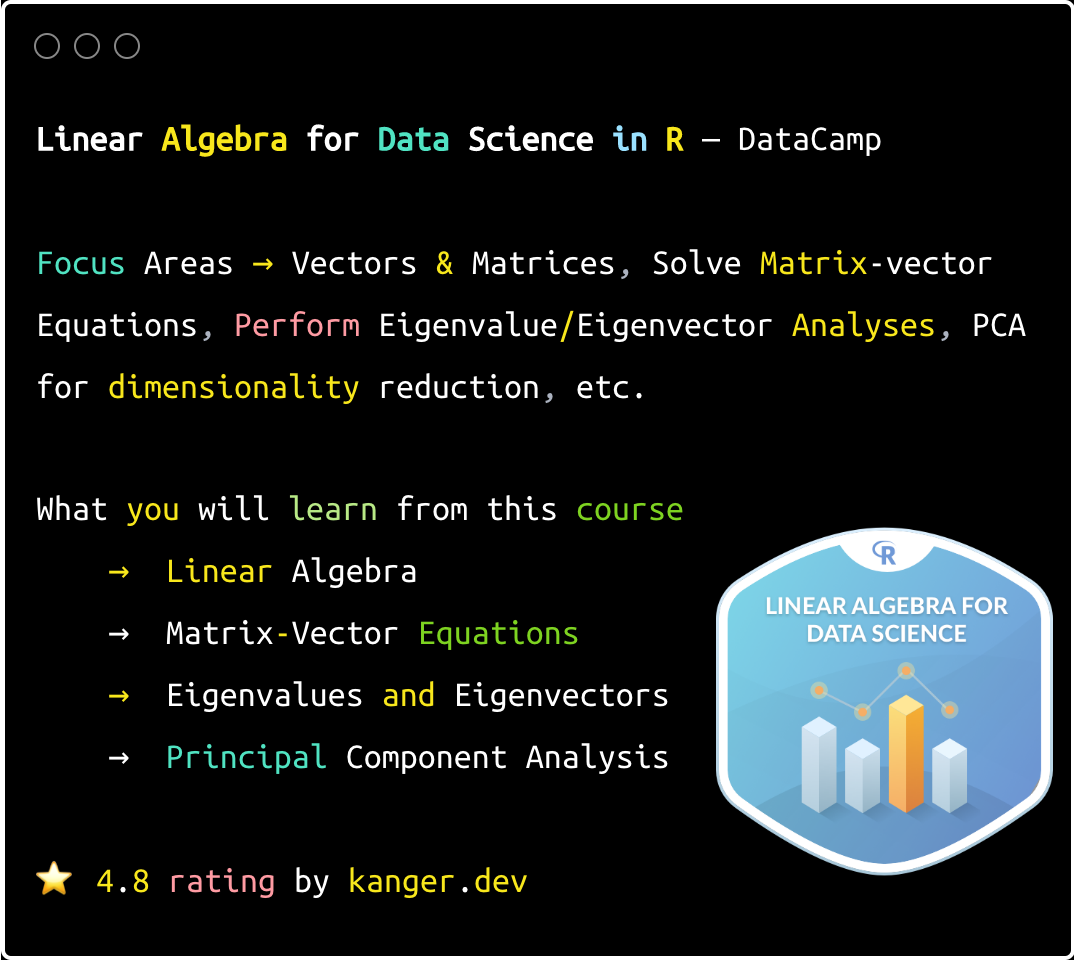Is it right for you?

This interactive course is suitable for learners with experience in R programming and basic knowledge of high-school-level mathematics. It aims to teach data science beginners the skills they lack to learn advanced topics. By the end, you'll have gained a high-level familiarity with essential linear algebra concepts commonly used in data science.

Linear Algebra for Data Science in R

### Mathematics for Machine Learning: Linear Algebra

The renowned Imperial College London - Mathematics for Machine Learning: Linear Algebra course is one of the best programs that will equip you with practical skills. This 19-hour course is jam-packed with lessons and exercises to help you develop an intuitive understanding of linear algebra concepts such as vectors and matrices, basis (linear algebra), eigenvalues and eigenvectors, and transformation matrix, among others.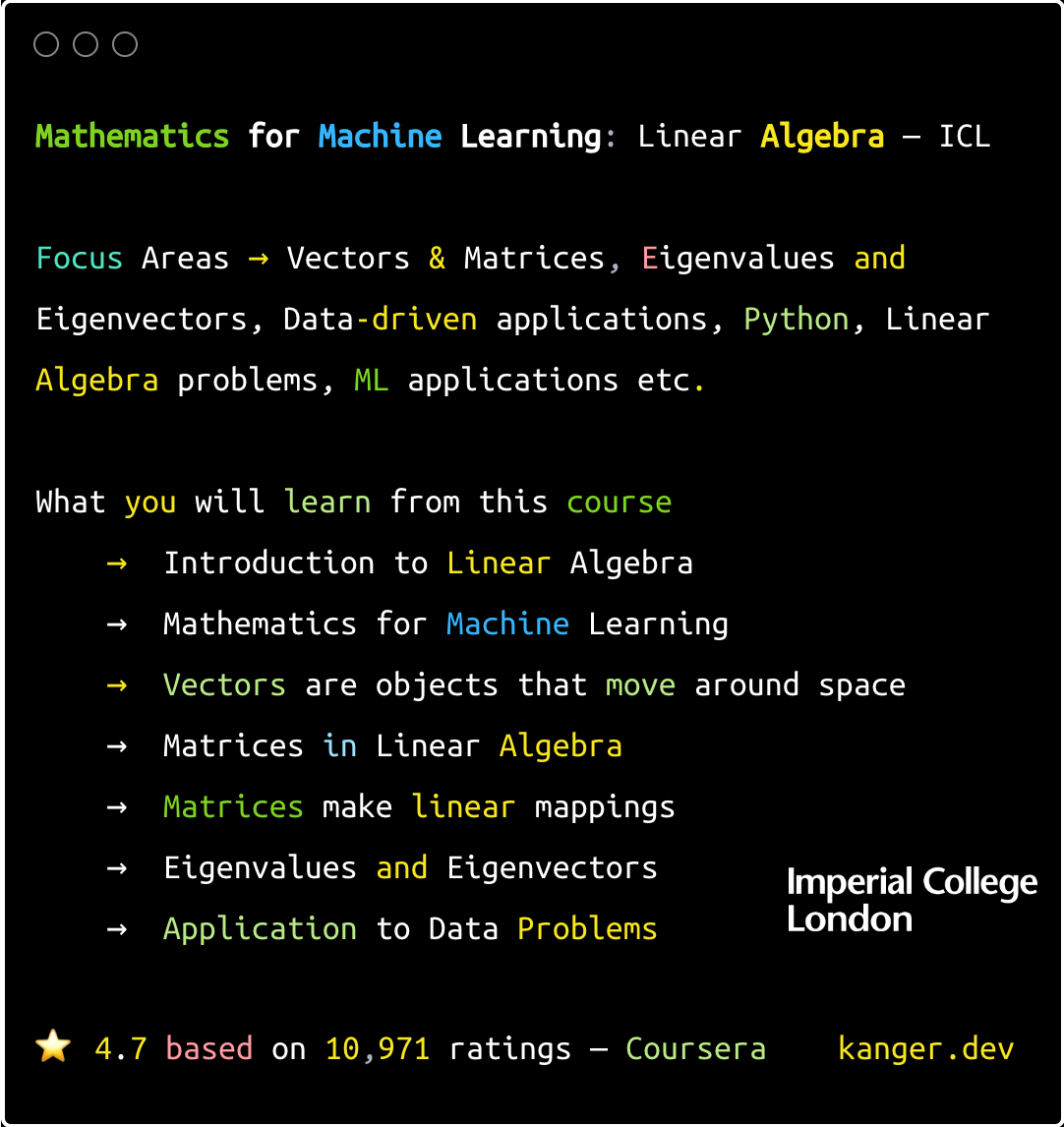Is it right for you?

This program is suitable for mid-level Python programmers to apply linear algebra to analyze data, create, and make predictions. You'll receive a university-backed curriculum and a formal qualification in the specialization program that includes Multivariate Calculus and PCA courses for machine learning.

Mathematics for Machine Learning: Linear Algebra

### Linear Algebra for Data Science Using Python Specialization

Another top source is Howard University, known for its skill-specific content. Its Linear Algebra Specialization is a good introduction aimed at Python beginners, building upon their previous mathematical foundations for data science. The course covers fundamentals essential for analyzing large datasets, making you better equipped for the task.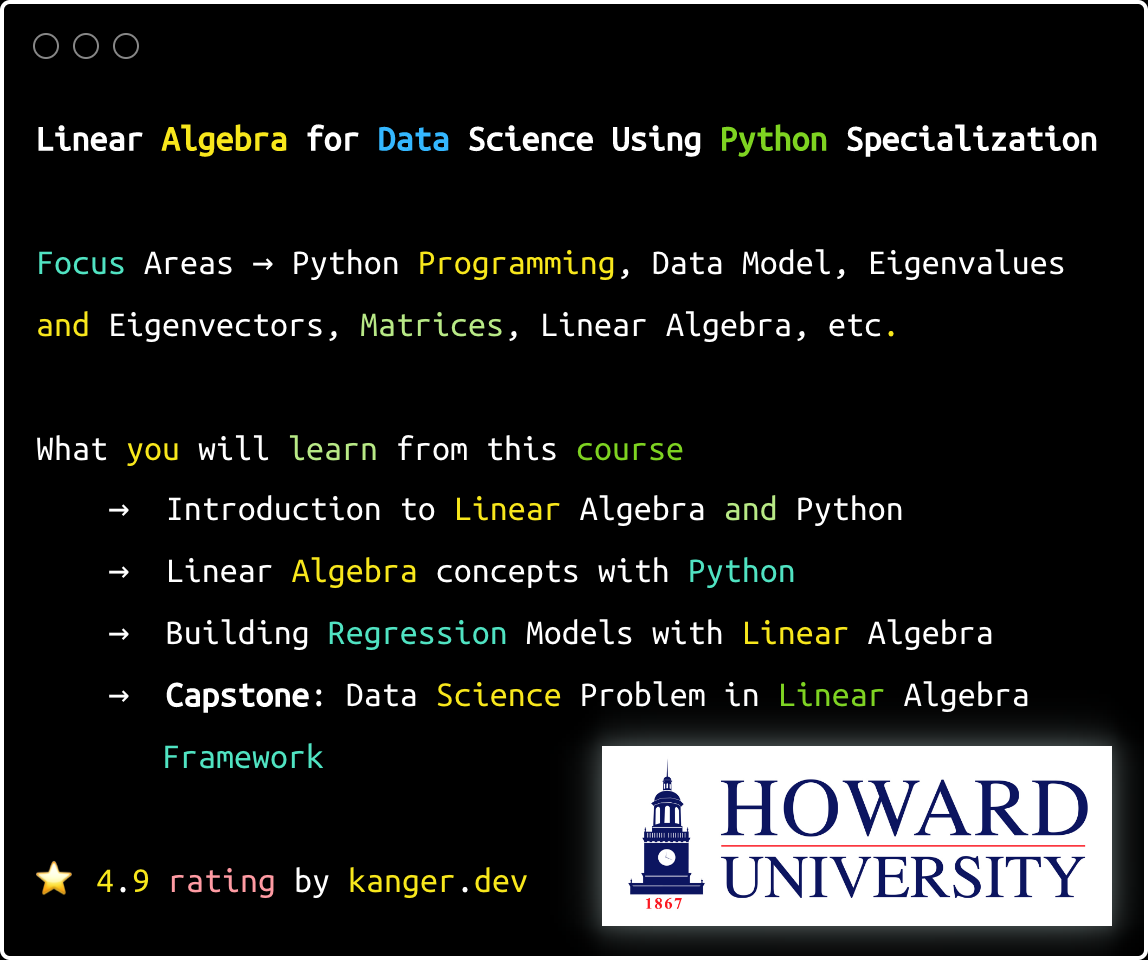Is it right for you?

This program is a popular choice for Python beginners who wish to gain a well-rounded foundation in algebra using Python. It assumes mathematical intuition and knowledge of calculus. By the end, you will be able to employ linear regression models to solve problems, apply your skills to analyze data, and make predictions based on a linear regression model.

Linear Algebra for Data Science Using Python Specialization

### Applying Linear Algebra with R

Applying Linear Algebra with R Programming is a mathematically intense course for learning the math behind predictive models. You'll learn basic matrix arithmetic, advanced matrix mathematics, linear regression, and other machine learning topics. The course explores the underlying machine learning process to help students develop an algebraic fluency in R programming.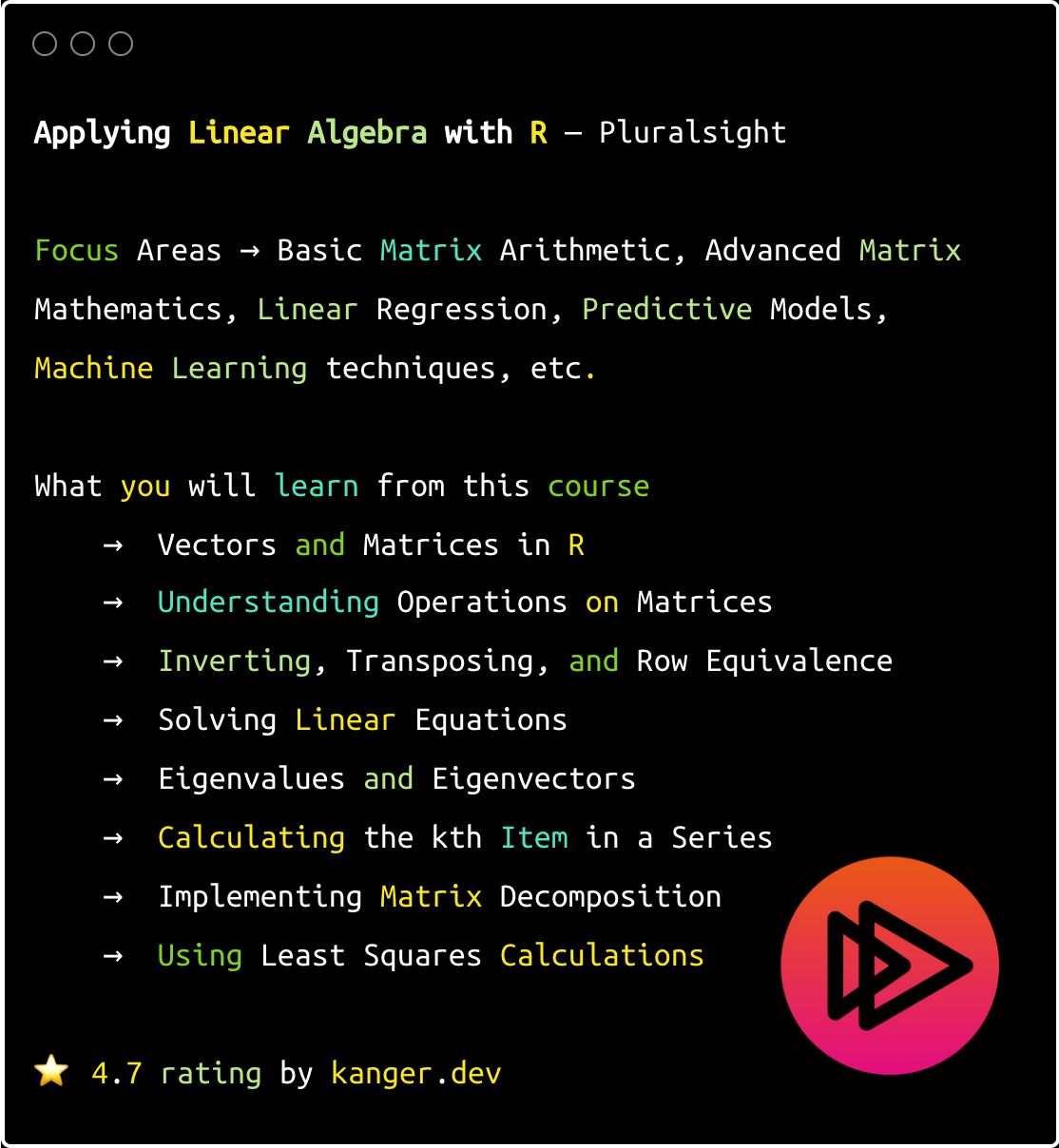Is it right for you?

The course is best suited for aspiring ML researchers who have an aptitude for problem-solving and R programming skills. It is also suitable for professionals in adjacent fields such as data science, deep learning, AI, and more. After completing the course, you will have the skills to implement machine learning techniques and the fundamental knowledge to learn advanced topics such as generalized linear models.

Applying Linear Algebra with R

Still wondering whether learning Linear Algebra program is of any value? Read on for answers to frequently asked questions about Linear Algebra for Data Science and Machine Learning courses.

### Is Linear Algebra fundamental for Data Science?

Short answer is yes, it is foundational for data science. Linear algebra is the language of algorithms that powers diverse data science algorithms and popular applications like natural language processing and computer vision. If you're not aiming for low-hanging fruit, consider linear algebra a must-know subject in data science for a rewarding career.

### Is Linear Algebra Hard to Learn?

The basics are not very difficult, but it can be challenging for absolute beginners. The obstacle for many learners is finding the right course that teaches terms and definitions, as well as how to determine the type of calculation and analysis needed to obtain the required results. It also depends on whether you are learning Linear Algebra for data science or machine learning.

### Is Linear Algebra Necessary for Data Science?

To become a competent Machine Learning Engineer/ Scientist or a Data Scientist, you need to know linear algebra and optimization theory. Understanding linear algebra is a fundamental aspect of machine learning (ML). Areas of mathematics essential for machine learning, such as calculus, require prior knowledge of linear algebra, which will help you understand ML to a greater extent.

### What is the best Linear Algebra Course for Data Science?

These Linear Algebra courses are designed to take you from a total novice to a talented ML practitioner, focusing on both Linear Algebra and its practical applications. It is important to know which math you need to learn and become equipped with a working knowledge for studying advanced Machine Learning topics. You will learn the fundamentals with programming (Python/R) to become a trained practitioner, but you should not expect to become a scientist overnight. It takes burning some midnight oil.

### What Else Can You do to Learn Algebra for Data Science?

In addition to a Linear Algebra course, you can improve your algebra skills by watching YouTube tutorials, practicing with books, exploring different tools, and reading blogs. Check out our Math Stack to discover resources and introductory study guides that explain how to learn in-demand math skills.

CLOSING NOTE

There are numerous online Linear Algebra courses available, each with its own strengths and weaknesses. Before starting an immersive Data Science or Machine Learning Certification, take a few of these Linear Algebra classes to give yourself a firm foundation. Familiarizing yourself with the mathematics, algorithms, machine learning concepts, and different tools and math that researchers use will help you start strongly.

Banner Image Credits:

Contributors
Editors
No spam. In-depth analysis, expert opinions, startup perks, and resources to bootstrap your growth.
Oops! Something went wrong while submitting the form.

## Trending Stacks

Please bear with us, an update is coming soon.
Data Engineering
Master Data Engineering with these powerful tools, techniques, and resources.
Data Science
Discover a wealth of data science resources to elevate your analytical skills.
Cloud Computing
Elevate your cloud computing skills with up-to-date resources.